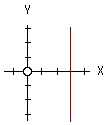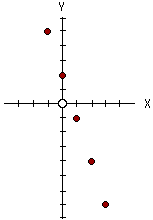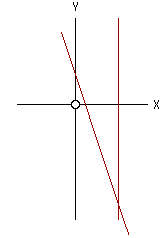David

How do you graph the equation x=3 on a x,y graph?

Also how do you graph f(x)=-3x + 2 on the same graph?

Hi David

The x,y graph of some condition or expression is the collection of points (x,y) that satisfy the condition.
 In your first question the graph is the collection of points, (x,y) that satisfy x=3, that is the set of points of the form (3,y). Since there is no condition that restricts y it can be any number and the graph is the vertical line three units to the right of the Y-axis.In your second problem, f(x) = -3x + 2 or y = -3x + 2, the graph is the set of points (x,y) that satisfy y = -3x + 2, i.e. the set of points of the form (x,-3x + 2). To plot graph you might construct a table of values and then plot the points.
x y = -3 x + 2
02
1-1
2-4
3-7
-15Joining these points, which lie on a line, and putting the two graphs together you getI hope this helps

Harley and Maxine

Go to Math Central